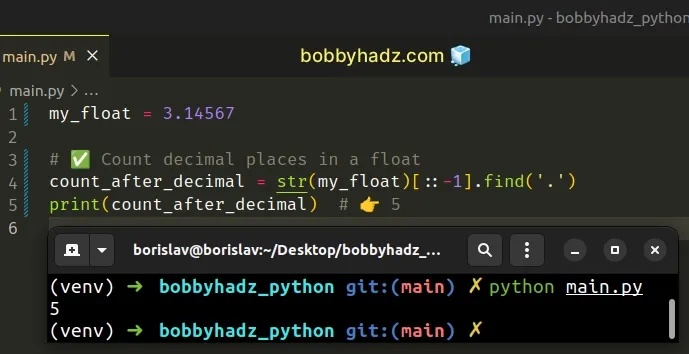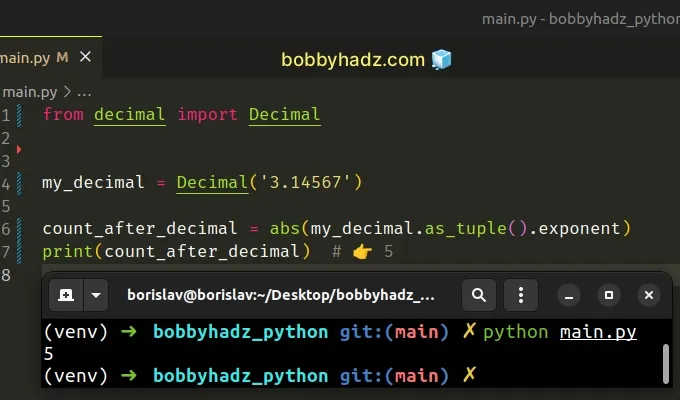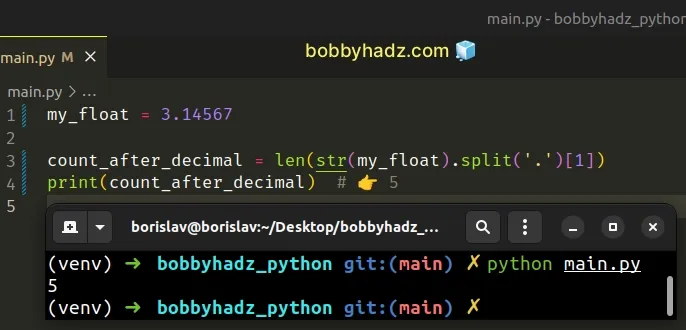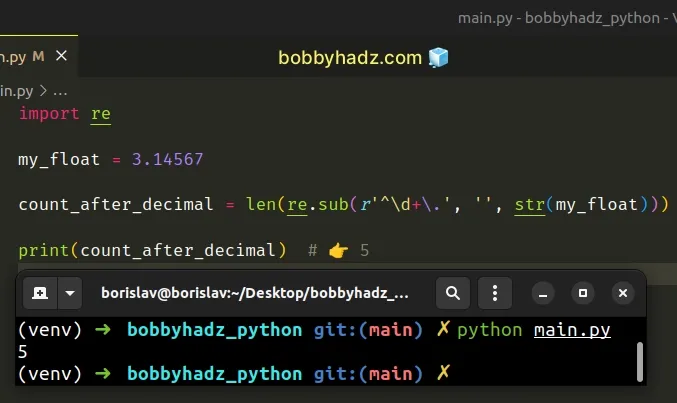# Count the decimal places of a Float in PythonLast updated: Feb 21, 2023
4 min## #Count the decimal places of a Float in Python

To get the number of digits after the decimal point:

1. Use the `str()` class to convert the number to a string.
2. Use string slicing to reverse the string.
3. Use the `str.find()` method to find the index of the period.
main.py
```Copied!```my_float = 3.14567

# ✅ Count decimal places in a float
count_after_decimal = str(my_float)[::-1].find('.')
print(count_after_decimal)  # 👉️ 5
``````The example counts the number of digits after the decimal in a floating-point number.

We used the `str()` class to convert the float to a string and reversed the string using string slicing.
main.py
```Copied!```my_float = 3.14567

print(str(my_float)[::-1]) # 👉️ 76541.3
``````

The syntax for string slicing is `my_str[start:stop:step]`.

We specified a value for the `step` of `-1` to reverse the string.

The last step is to use the `str.find()` method to get the index of the period.

main.py
```Copied!```my_float = 3.14567

count_after_decimal = str(my_float)[::-1].find('.')
print(count_after_decimal)  # 👉️ 5
``````
Python indexes are zero-based, so the first character in a string has an index of `0`, and the last character has an index of `-1` or `len(my_str) - 1`.

Since indexes are zero-based, the index of the period is equal to the number of decimal places in the number.

Note that you might run into unexpected rounding issues when using floating-point numbers.

main.py
```Copied!```my_float = 3.14567834 - 1.3123
print(my_float)  # 👉️ 1.8333783399999999

count_after_decimal = str(my_float)[::-1].find('.')
print(count_after_decimal)  # 👉️ 16
``````

## #Count the decimal places of a Decimal object in Python

You can use the `exponent` attribute to get the number of digits after the decimal point if you use the `Decimal` class.

main.py
```Copied!```from decimal import Decimal

my_decimal = Decimal('3.14567')

count_after_decimal = abs(my_decimal.as_tuple().exponent)
print(count_after_decimal)  # 👉️ 5
``````The as_tuple method returns a named tuple representation of the number.

main.py
```Copied!```my_decimal = Decimal('3.14567')

print(my_decimal.as_tuple())

print(my_decimal.as_tuple().sign)  # 👉️ 0
print(my_decimal.as_tuple().digits)  # 👉️ (3, 1, 4, 5, 6, 7)
print(my_decimal.as_tuple().exponent)  # 👉️ -5
``````

The named tuple has `sign`, `digits` and `exponent` attributes.

To get the number of digits after the decimal, we have to change the sign of the `exponent` value, so we passed the result to the `abs()` function.

main.py
```Copied!```from decimal import Decimal

my_decimal = Decimal('3.14567')

count_after_decimal = abs(my_decimal.as_tuple().exponent)
print(count_after_decimal)  # 👉️ 5
``````

The abs function returns the absolute value of a number. In other words, if the number is positive, the number is returned, and if the number is negative, the negation of the number is returned.

main.py
```Copied!```print(abs(-50))  # 👉️ 50
print(abs(50))  # 👉️ 50
``````

## #Count the decimal places of a Float using split()

You can also use the `split()` method to count the decimal places of a floating-point number.

main.py
```Copied!```my_float = 3.14567

count_after_decimal = len(str(my_float).split('.'))
print(count_after_decimal) # 👉️ 5
``````We used the str() class to convert the float to a string and split the string on the period.

main.py
```Copied!```my_float = 3.14567

print(str(my_float).split('.')) # 👉️ ['3', '14567']
``````

The second element in the list is the decimal part.

Python indexes are zero-based, so the first item in a list has an index of `0`, and the last item has an index of `-1` or `len(a_list) - 1`.

We accessed the list at index `1` and used the `len()` function to get the count of the decimal places.

The len() function returns the length (the number of items) of an object.

## #Count the decimal places of a Float using re.sub()

You can also use the `re.sub()` method to count the decimal places in a float.

main.py
```Copied!```import re

my_float = 3.14567

count_after_decimal = len(re.sub(r'^\d+\.', '', str(my_float)))

print(count_after_decimal)  # 👉️ 5
``````The re.sub method returns a new string that is obtained by replacing the occurrences of the pattern with the provided replacement.

The first argument we passed to the method is a regular expression.

The `\d` character matches the digits `[0-9]` (and many other digit characters).

The plus `+` causes the regular expression to match 1 or more repetitions of the preceding character (the digit).

We used a backslash to escape the period because periods have a special meaning in regular expressions.

In its entirety, the regular expression matches one or more digits at the start of the string followed by a period and removes the characters by replacing them with an empty string.

The last step is to use the `len()` function to count the number of digits after the decimal.